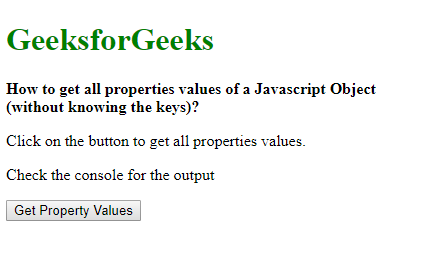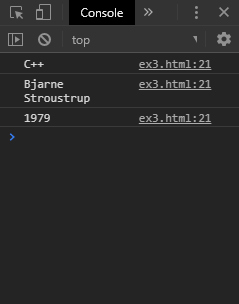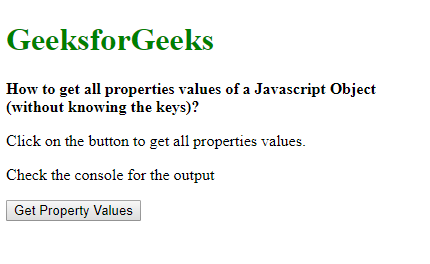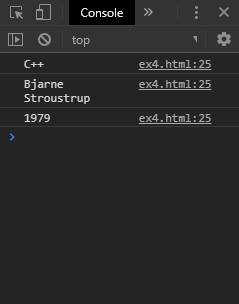# How to get all property values of a JavaScript Object (without knowing the keys) ?

Method 1: Using Object.values() Method: The Object.values() method is used to return an array of the object’s own enumerable property values. The array can be looped using a for-loop to get all the values of the object. Therefore, the keys are not required to be known to get all the property values.

Syntax:

 `let valuesArray = Object.values(exampleObj); ` ` `  `for` `(let value of valuesArray) { ` `    ``console.log(value); ` `} `

Example:

 ` ` `<``html``> ` `<``head``> ` `  ``<``title``> ` `    ``How to get all properties ` `    ``values of a Javascript Object ` `    ``(without knowing the keys)? ` `  `` ` ` ` `<``body``> ` `  ``<``h1` `style``=``"color: green"``> ` `    ``GeeksforGeeks ` `  `` ` `  ``<``b``> ` `    ``How to get all properties ` `    ``values of a Javascript Object ` `    ``(without knowing the keys)? ` `  `` ` `  ``<``p``> ` `    ``Click on the button to get all ` `    ``properties values. ` `  `` ` `  ``<``p``> ` `    ``Check the console for the output ` `  `` ` `  ``<``button` `onclick``=``"getValues()"``> ` `    ``Get Property Values ` `  `` ` `  ``<``script` `type``=``"text/javascript"``> ` `    ``function getValues() { ` `      ``let exampleObj = { ` `        ``language: "C++", ` `        ``designedby: "Bjarne Stroustrup", ` `        ``year: "1979" ` `      ``}; ` ` `  `      ``let valuesArray = Object.values(exampleObj); ` ` `  `      ``for (let value of valuesArray) { ` `        ``console.log(value); ` `      ``} ` `    ``} ` `  `` ` ` ` ` `

Output:

• Before clicking the button:• After clicking the button:Method 2: Extracting the keys to access the properties: The Object.keys() method is used to return an array of objects own enumerable property names. The forEach() method is used on this array to access each of the keys. The value of each property can be accessed using the keys with an array notation of the object.
Therefore, the keys are not required to be known beforehand to get all the property values.

Syntax:

 `let objKeys = Object.keys(exampleObj); ` ` `  `objKeys.forEach(key => { ` `    ``let value = exampleObj[key]; ` ` `  `    ``console.log(value); ` `}); `

Example:

 ` ` `<``html``> ` `<``head``> ` `   ``<``title``> ` `    ``How to get all properties ` `    ``values of a Javascript Object ` `    ``(without knowing the keys)? ` `  `` ` ` ` `<``body``> ` `  ``<``h1` `style``=``"color: green"``> ` `    ``GeeksforGeeks ` `  `` ` `  ``<``b``> ` `    ``How to get all properties ` `    ``values of a Javascript Object ` `    ``(without knowing the keys)? ` `  `` ` `  ``<``p``> ` `    ``Click on the button to get all ` `    ``properties values. ` `  `` ` `  ``<``p``> ` `    ``Check the console for the output ` `  `` ` `  ``<``button` `onclick``=``"getValues()"``> ` `    ``Get Property Values ` `  `` ` `  ``<``script` `type``=``"text/javascript"``> ` `    ``function getValues() { ` `      ``let exampleObj = { ` `          ``language: "C++", ` `          ``designedby: "Bjarne Stroustrup", ` `          ``year: "1979" ` `        ``}; ` ` `  `      ``let objKeys = Object.keys(exampleObj); ` ` `  `      ``objKeys.forEach(key => { ` `        ``let value = exampleObj[key]; ` ` `  `        ``console.log(value); ` `      ``}); ` `    ``} ` `  `` ` ` ` ` `

Output:

• Before clicking the button:• After clicking the button:My Personal Notes arrow_drop_upCheck out this Author's contributed articles.

If you like GeeksforGeeks and would like to contribute, you can also write an article using contribute.geeksforgeeks.org or mail your article to contribute@geeksforgeeks.org. See your article appearing on the GeeksforGeeks main page and help other Geeks.

Please Improve this article if you find anything incorrect by clicking on the "Improve Article" button below.